Gradient of a multivariate, scalar-valued function using the forward difference approximation.

## Syntax

g = fgradient(f,x0)


## Description

g = fgradient(f,x0) numerically evaluates the gradient of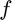with respect to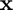at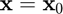using the forward difference approximation with a default relative step size of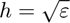, where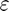is the machine zero.

g = fgradient(f,x0,h) numerically evaluates the gradient ofwith respect toatusing the forward difference approximation with a user-specified relative step size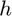.

## Input/Output Parameters

 Variable Symbol Description Format Input f multivariate, scalar-valued function () 1×1function_handle x0 evaluation point n×1double h (OPTIONAL) relative step size (defaults to ) 1×1double Output g gradient of with respect to , evaluated at n×1double

## Note

• This function requires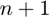evaluations of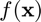.

## Example

Approximate the gradient of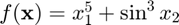at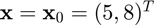using the fgradient function, and compare the result to the true result of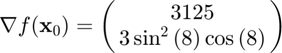f = @(x) x(1)^5+sin(x(2))^3;
x0 = [5;8];

g =

1.0e+03 *

3.1250
-0.0004



Calculating the error,

error = g-[3125;3*sin(8)^2*cos(8)]

error =

1.0e-03 *

0.1119
-0.0004